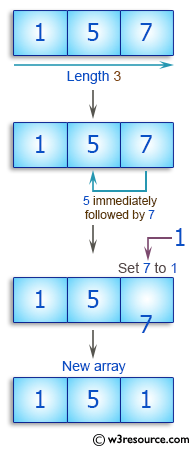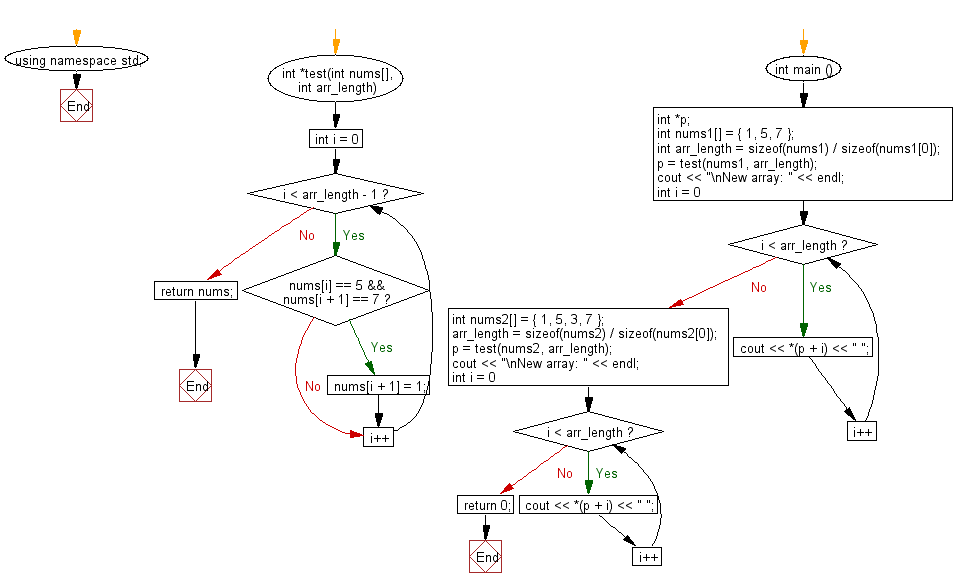﻿ C++ : Check an array, length 3 and create a new array# C++ Exercises: Check a given array of integers, length 3 and create a new array

## C++ Basic Algorithm: Exercise-92 with Solution

Write a C++ program to check a given array of integers, length 3 and create a new array. If there is a 5 in the given array immediately followed by a 7 then set 7 to 1.

Sample Solution:

C++ Code :

``````#include <iostream>
using namespace std;

int *test(int nums[], int arr_length) {

for (int i = 0; i < arr_length - 1; i++)
{
if (nums[i] == 5 && nums[i + 1] == 7)
nums[i + 1] = 1;
}
return nums;
}

int main () {
int *p;
int nums1[] = { 1, 5, 7 };
int arr_length = sizeof(nums1) / sizeof(nums1);

p = test(nums1, arr_length);

cout << "\nNew array: " << endl;
for ( int i = 0; i < arr_length; i++ ) {
cout << *(p + i) << " ";
}
int nums2[] = { 1, 5, 3, 7 };
arr_length = sizeof(nums2) / sizeof(nums2);

p = test(nums2, arr_length);

cout << "\nNew array: " << endl;
for ( int i = 0; i < arr_length; i++ ) {
cout << *(p + i) << " ";
}
return 0;
}
``````

Sample Output:

```New array:
1 5 1
New array:
1 5 3 7
```

Pictorial Presentation:Flowchart:C++ Code Editor:

Contribute your code and comments through Disqus.

What is the difficulty level of this exercise?

﻿